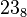# Base (mathematics) facts for kids

Kids Encyclopedia Facts

In mathematics, a base or radix is the number of different digits or combination of digits and letters that a system of counting uses to represent numbers. For example, the most common base used today is the decimal system. Because "dec" means 10, it uses the 10 digits from 0 to 9. Most people think that we most often use base 10 because we have 10 fingers.

A base is usually a whole number bigger than 1, although non-integer bases are also mathematically possible. The base of a number may be written next to the number: for instance,$23_8$ means 23 in base 8 (which is equal to 19 in base 10).

## In computers

Different bases are often used in computers. Binary (base 2) is used because at the most simple level, computers can only deal with 0s and 1s. Hexadecimal (base 16) is used because of how computers group binary digits together. Every four binary digits turn into one hexadecimal digit when changing between them. Because there are more than 10 digits in hexadecimal, the six digits after 9 are shown as A, B, C, D, E, and F.

## Measurement

The oldest systems of counting used base one. Making marks on a wall, using one mark for each item counted is an example of unary counting. Some old systems of measurement use the duodecimal radix (base twelve). This is shown in English, as there are words such as dozen (12) and gross (144 = 12×12), and lengths such as feet (12 inches). Angle measurement often uses a system adapted from the Babylonian numerals with base 60.

## Writing bases

When typing a base, the small number indicating the base is usually in base ten. This is because if the radix were written in its own base, it would always be "10," so there would be no way of knowing what base it was supposed to be in.

## Numbers in different bases

Here are some examples of how some numbers are written in different bases, compared to decimals:

Decimal (Base 10) Binary (Base 2) Octal (Base 8) Undecimal (Base 11) Hexadecimal (Base 16) Senary (Base 6) Unary (Base 1)
1 1 1 1 1 1 1
2 10 2 2 2 2 11
3 11 3 3 3 3 111
4 100 4 4 4 4 1111
5 101 5 5 5 5 11111
6 110 6 6 6 10 111111
7 111 7 7 7 11 1111111
8 1000 10 8 8 12 11111111
9 1001 11 9 9 13 111111111
10 1010 12 A A 14 1111111111
11 1011 13 10 B 15 11111111111
12 1100 14 11 C 20 111111111111
13 1101 15 12 D 21 1111111111111
14 1110 16 13 E 22 11111111111111
15 1111 17 14 F 23 111111111111111
16 10000 20 15 10 24 1111111111111111Base (mathematics) Facts for Kids. Kiddle Encyclopedia.